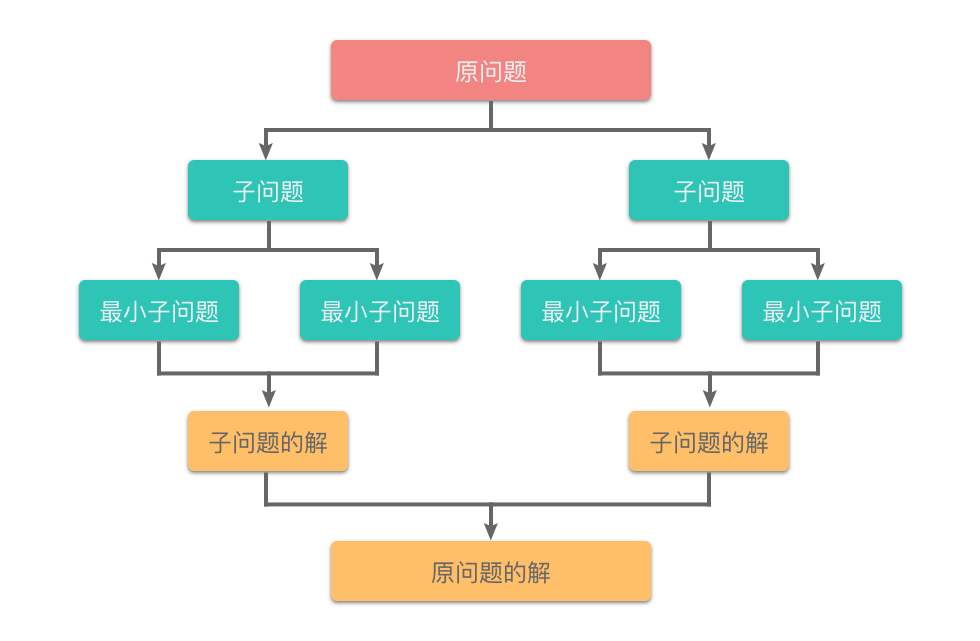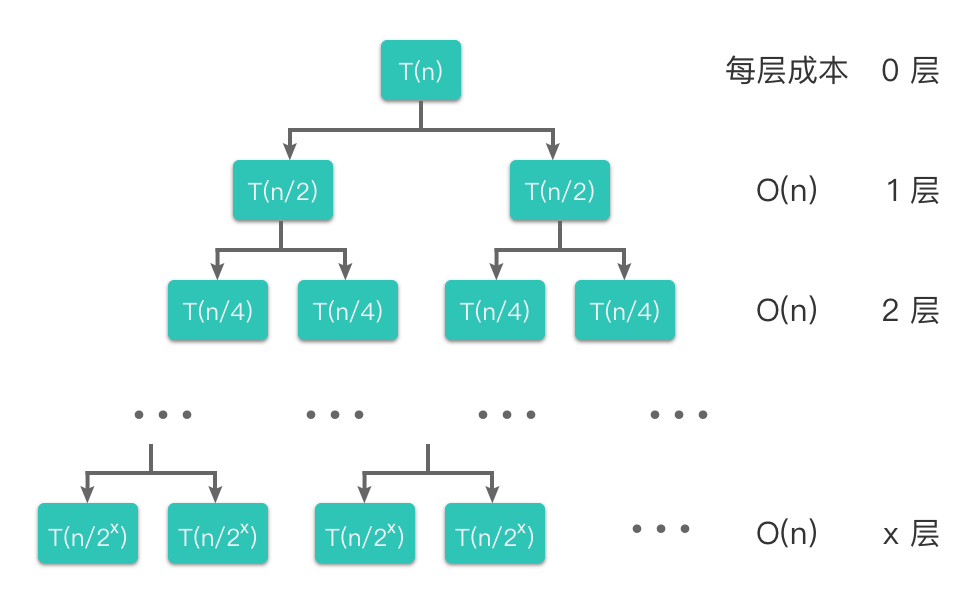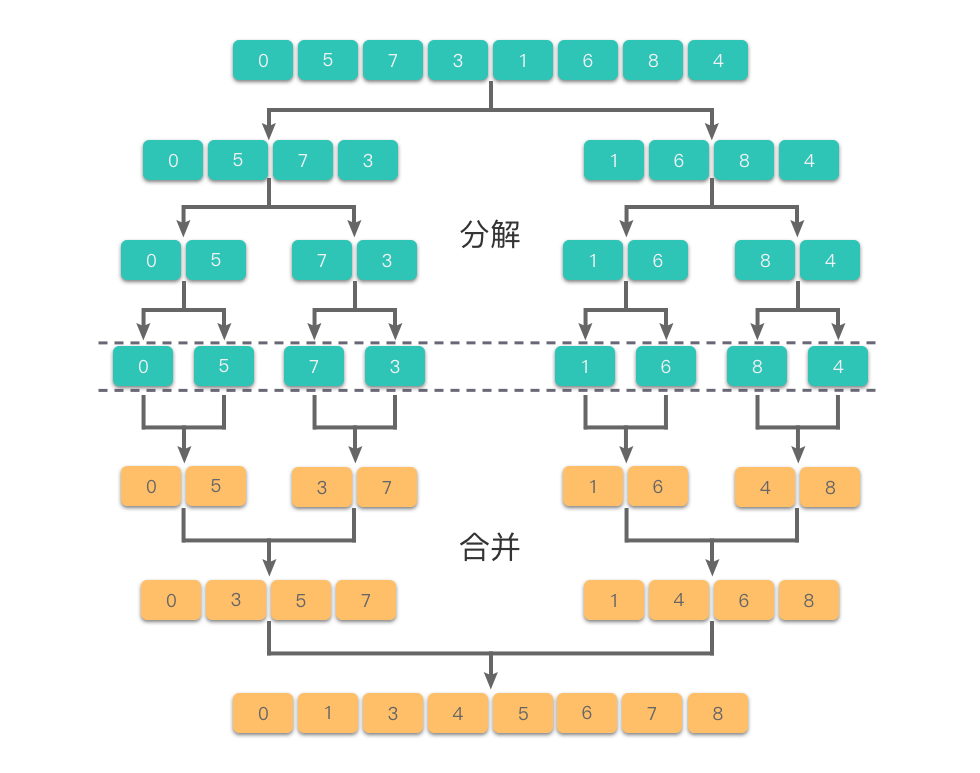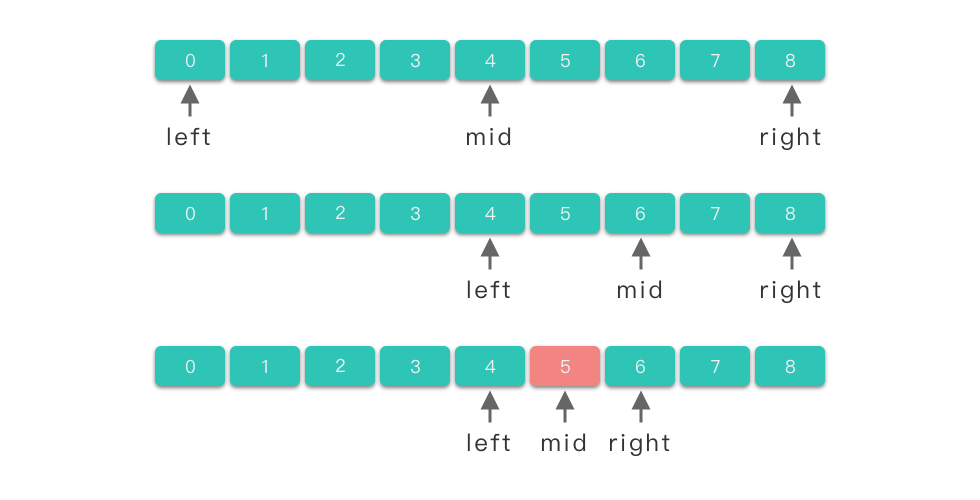01.分治算法知识

# 分治算法知识 #

## 1. 分治算法简介 #

### 1.1 分治算法的定义 #### 1.2 分治算法和递归算法的异同 #### 1.3 分治算法的适用条件 #

1. 原问题可以分解为若干个规模较小的相同子问题。
2. 分解出来的子问题可以独立求解，即子问题之间不包含公共的子子问题。
3. 具有分解的终止条件，也就是说当问题的规模足够小时，能够用较简单的方法解决。
4. 子问题的解可以合并为原问题的解，并且合并操作的复杂度不能太高，否则就无法起到减少算法总体复杂度的效果了。

## 2. 分治算法的基本步骤 #

1. 分解：把要解决的问题分解为成若干个规模较小、相对独立、与原问题形式相同的子问题。
2. 求解：递归求解各个子问题。
3. 合并：按照原问题的要求，将子问题的解逐层合并构成原问题的解。

  1 2 3 4 5 6 7 8 9 10 11 12  def divide_and_conquer(problem): # problem 为问题规模 if problem < d: # 当问题规模足够小时，直接解决该问题 return solove(); # 直接求解 k_problems = divide(problem) # 将问题分解为 k 个相同形式的子问题 res = [0 for _ in range(k)] # res 用来保存 k 个子问题的解 for k_problem in k_problems: res[i] = divide_and_conquer(k_problem) # 递归的求解 k 个子问题 ans = merge(res) # 合并 k 个子问题的解 return ans # 返回原问题的解 

## 3. 分治算法的复杂度分析 #

$T(n) = \begin{cases} \begin{array} \ \Theta{(1)} & n = 1 \cr a * T(n/b) + f(n) & n > 1 \end{array} \end{cases}$

### 3.1 递推求解法 #

$T(n) = \begin{cases} \begin{array} \ O{(1)} & n = 1 \cr 2T(n/2) + O(n) & n > 1 \end{array} \end{cases}$

\begin{align} T(n) & = 2T(n/2) + O(n) \cr & = 2(2T(n / 4) + O(n/2)) + O(n) \cr & = 4T(n/4) + 2O(n) \cr & = 8T(n/8) + 3O(n) \cr & = …… \cr & = 2^x \times T(n/2^x) + x \times O(n) \end{align}

\begin{align} T(n) & = n \times T(1) + \log_2n \times O(n) \cr & = n + \log_2n \times O(n) \cr & = O(n \times \log_2n) \end{align}

### 3.2 递归树法 #

$时间复杂度 = 叶子数 * T(1) + 成本和 = 2^x \times T(1) + x \times O(n)$。

$T(n) = \begin{cases} \begin{array} \ O{(1)} & n = 1 \cr 2T(n/2) + O(n) & n > 1 \end{array} \end{cases}$## 4. 分治算法的应用 #

### 4.1 归并排序 #

#### 4.1.2 题目大意 #

• $1 \le nums.length \le 5 * 10^4$。
• $-5 * 10^4 \le nums[i] \le 5 * 10^4$。

 1 2  输入 nums = [5,2,3,1] 输出 [1,2,3,5] 

#### 4.1.3 解题思路 #

1. 分解：将待排序序列中的 n 个元素分解为左右两个各包含 n / 2 个元素的子序列。
2. 求解：递归将子序列进行分解和排序，直到所有子序列长度为 1
3. 合并：把当前序列组中有序子序列逐层向上，进行两两合并。#### 4.1.4 代码 #

  1 2 3 4 5 6 7 8 9 10 11 12 13 14 15 16 17 18 19 20 21 22 23 24 25 26  class Solution: def merge(self, left_arr, right_arr): # 合并 arr = [] while left_arr and right_arr: # 将两个排序数组中较小元素依次插入到结果数组中 if left_arr <= right_arr: arr.append(left_arr.pop(0)) else: arr.append(right_arr.pop(0)) while left_arr: # 如果左子序列有剩余元素，则将其插入到结果数组中 arr.append(left_arr.pop(0)) while right_arr: # 如果右子序列有剩余元素，则将其插入到结果数组中 arr.append(right_arr.pop(0)) return arr # 返回排好序的结果数组 def mergeSort(self, arr): # 分解 if len(arr) <= 1: # 数组元素个数小于等于 1 时，直接返回原数组 return arr mid = len(arr) // 2 # 将数组从中间位置分为左右两个数组。 left_arr = self.mergeSort(arr[0: mid]) # 递归将左子序列进行分解和排序 right_arr = self.mergeSort(arr[mid:]) # 递归将右子序列进行分解和排序 return self.merge(left_arr, right_arr) # 把当前序列组中有序子序列逐层向上，进行两两合并。 def sortArray(self, nums: List[int]) -> List[int]: return self.mergeSort(nums) 

### 4.2 二分查找 #

#### 4.2.2 题目大意 #

• 假设 nums 中的所有元素是不重复的。
• n 将在 [1, 10000]之间。
• $-9999 \le nums[i] \le 9999$。

 1 2 3  输入 nums = [-1,0,3,5,9,12], target = 9 输出 4 解释 9 出现在 nums 中并且下标为 4 

#### 4.2.3 解题思路 #

1. 分解：将数组的 n 个元素分解为左右两个各包含 n / 2 个元素的子序列。
2. 求解：取中间元素 nums[mid]target 相比。
1. 如果相等，则找到该元素；
2. 如果 nums[mid] < target，则递归在左子序列中进行二分查找。
3. 如果 nums[mid] > target，则递归在右子序列中进行二分查找。#### 4.2.4 代码 #

  1 2 3 4 5 6 7 8 9 10 11 12 13 14 15 16  class Solution: def search(self, nums: List[int], target: int) -> int: left = 0 right = len(nums) - 1 # 在区间 [left, right] 内查找 target while left < right: # 取区间中间节点 mid = left + (right - left) // 2 # nums[mid] 小于目标值，排除掉不可能区间 [left, mid]，在 [mid + 1, right] 中继续搜索 if nums[mid] < target: left = mid + 1 # nums[mid] 大于等于目标值，目标元素可能在 [left, mid] 中，在 [left, mid] 中继续搜索 else: right = mid # 判断区间剩余元素是否为目标元素，不是则返回 -1 return left if nums[left] == target else -1